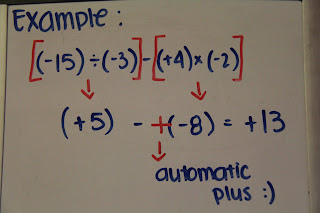## Wednesday, March 23, 2011

### Jocelle’s Great Big Book of Integers

Chapter 1 : Grade 7 Integer Review

The Sign Rule :

Chapter 3 : Dividing Integers

Example:

The sign rule for division is the same as the sign rule for multiplication, addition, and subtraction.

6 ÷ 2 = 3 There are no negative signs in the question, therefore the answer it positive.

-6 ÷ (-2) = 3 There is an even number of negative signs in the question, so the answer is positive.

(-6) ÷ 2 = -3 There is an odd number (one) of negative signs in the question, therefore the answer is negative.

6 ÷ (-2) = -3 There is one negative sign in the question, therefore the answer is negative.

Chapter 4 : Order of Operations with Integers

Use B.E.D.M.A.S. to solve equations except "E"